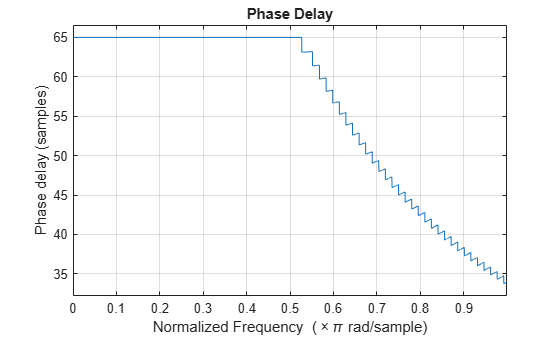# phasedelay

Phase delay response of discrete-time filter System object

## Syntax

```[phi,w] = phasedelay(sysobj) [phi,w] = phasedelay(sysobj,n) [phi,w] = phasedelay(sysobj,Name,Value) phasedelay(sysobj) ```

## Description

```[phi,w] = phasedelay(sysobj)``` returns the phase delay `phi` of the filter System object™, `sysobj`, based on the current filter coefficients. The vector `w` contains the frequencies (in radians) at which the phase delay is evaluated.

The phase delay is evaluated at 8192 points equally spaced around the upper half of the unit circle.

```[phi,w] = phasedelay(sysobj,n)``` returns the phase delay of the filter System object at `n` points equally spaced around the upper half of the unit circle.

```[phi,w] = phasedelay(sysobj,Name,Value)``` returns the phase delay with additional options specified by one or more `Name,Value` pair arguments.

`phasedelay(sysobj)` plots the phase delay of the filter System object `sysobj` in the `fvtool`.

For information on more input options, refer to `phasedelay` in Signal Processing Toolbox™ documentation.

## Examples

collapse all

```Fs = 8000; Fcutoff = 2000; FIRFilt = dsp.FIRFilter('Numerator', fir1(130,Fcutoff/(Fs/2)));```

`phasedelay` computes the phase delay of the filter and displays it using fvtool

`phasedelay(FIRFilt);`## Input Arguments

collapse all

 `sysobj` Filter System object. The following Filter System objects are supported by this analysis function: `n` Number of samples. For an FIR filter where `n` is a power of two, the computation is done faster using FFTs. Default: 8192

### Name-Value Pair Arguments

Specify optional comma-separated pairs of `Name,Value` arguments. `Name` is the argument name and `Value` is the corresponding value. `Name` must appear inside quotes. You can specify several name and value pair arguments in any order as `Name1,Value1,...,NameN,ValueN`.

Specify the arithmetic used during analysis. When you specify `'double'` or `'single'`, the function performs double- or single-precision analysis. When you specify `'fixed'` , the arithmetic changes depending on the setting of the `CoefficientDataType` property and whether the System object is locked or unlocked.

When you do not specify the arithmetic for non-CIC structures, the function uses double-precision arithmetic if the filter System object is in an unlocked state. If the System object is locked, the function performs analysis based on the locked input data type. CIC structures only support fixed-point arithmetic.

## Output Arguments

 `phi` Phase delay vector of length `n`. If `n` is not specified, the function uses a default value of `8192`. `w` Frequency vector of length `n`, in radians/sample. `w` consists of `n` points equally spaced around the upper half of the unit circle (from 0 to π radians/sample). If `n` is not specified, the function uses a default value of `8192`.

## Algorithms

You can provide `fs`, the sampling frequency, as an input as well. `phasedelay` uses `fs` to calculate the delay response and plots the response to `fs`/2.

Watch now